# Equations

## Overview

The equation editor is one of the most versatile and powerful features of the Whiteboard. In this mode, you can write about beautifully formatted, dynamic equations.

## Selecting the Function tool

To begin with, select the Equation tool on the vertical toolbar on the left of the Whiteboard. The Equation tool looks like this:Once you click on the f(x) button, the lettering will turn blue.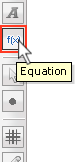Now, you're ready to type out on equation on the Board.

## Building Equations

With the f(x) button selected, DOUBLE CLICK anywhere on the Board. Once you do that, a gray box will appear with a blinking red cursor in it, and you're ready to go.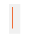To write out equations, simply start typing an equation in any format. The editor will automatically recognize which variable is independent, and which is dependent.

The equations can be formatted as

y=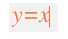f(x)=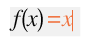or without an dependent variable at all (2x)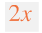Construct an equation

Once you decide you you want to construct your equation, type it out using the basic operations below:

Subtraction: -

Multiplication: *

Adding a / will instantly create a vertically formatted function, with a clear numerator and denominator. You can shift between the top and bottom lines of the equation by using your Up and Down Arrows.

Parentheses are very important in the equation editor. The editor has hidden parentheses built in so you can see the exact format of every equation you write, but you can add your own to shift the order of operation.

More advanced operations are listed below.

## Keyboard Shortcuts

There are a few important shortcuts that will enhance your functions. Getting to know them will help you make the most out of your equation tool.

Superscripts and Exponents

This handy shortcut will allow you to write out superscripts and exponents. While in Equation mode, simply press the ^ key (Shift+6). For example, you can type out x squared by typing out x (Shift+6) 2.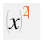Then, simply use your Down Arrow or Right Arrow to return to the main plain of the equation.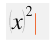Shift back to the superscript level with your Up Arrow or Left Arrow.

Subscripts

Just like the superscript shortcut, there's an easy way to drop your cursor down into subscript mode. While in Equation mode, simply press the _ key (Shift + -). For example, you can type out X (sub-two) by typing out X (Shift + -) 2.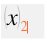Then, use your Up Arrow or Right Arrow to return to the main plain of the Equation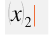Shift back to the subscript level with your Down Arrow or Left Arrow.

## Greek Letters and Mathematical Notation

Special Characters

The Whiteboard comes fully stocked with a whole range of symbols that work with the equation editor. The Secondary Toolbar contains a library of Greek, Math, and Language Characters that you can plug into your equations.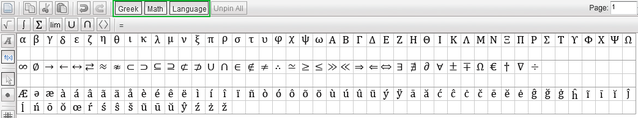Some important Greek letters and Math Symbols to remember for equations, with their corresponding keyboard shortcuts (in parentheses):

• Pi (pi#)
• Theta (theta#)
• Infinity (inf#)

Mathematical Notation

Once you've double clicked on the Whiteboard in equation mode, a new toolbar will appear underneath the Secondary Toolbar. This Mathematical Notation toolbar will look like this:Hover your mouse over each one to see what its keyboard shortcut will be. For example, the keyboard shortcut for Square Root is sqrt(: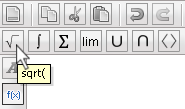From left to right, the buttons are as follows { shortcuts are in brackets }:

Square Roots { sqrt( }: Type out any expression and the radical will grow with the function until you add a closed parentheses )

Integral { int( }: Use your down and up arrows to set the lower and upper limits of the integral, respectively. Then, use your right arrow to begin typing the function. Do not specify the variable of integration (dx, for example), since the equation editor will automatically make this determination.

Sum { sum( }: Use your down and up arrows to set the lower and upper limits for your summation, then use your right arrow to enter in the formula you want to sum.

Limit { lim( }: As soon as you input a limit, your cursor will automatically drop to the left of the arrow underneath the limit. Here, you input the variable you want to use. Then, use your right arrow to shift into the location where you will input the limit. Use your right arrow again to begin inputting your function.

Union { union( }:

Intersection {inter( }

Angle Brackets { bracket( }

## Evaluating Equations

Evaluating Equations

**Note: The editor can only recognize functions of one variable. The editor will display, but not evaluate, multi-variable functions.